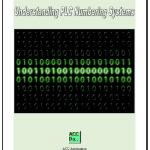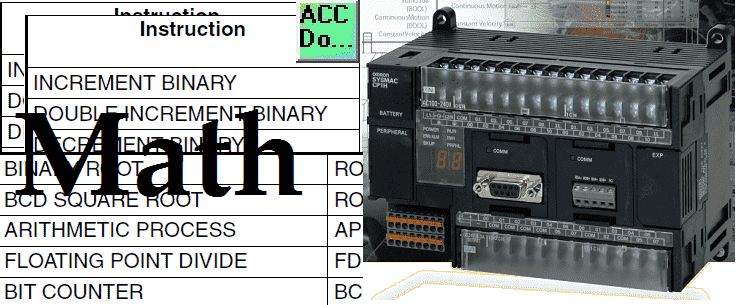# Omron CP1H Math Instructions

Programmable logic controllers are not typically known for their math ability. Modern PLC controllers now come with several math instructions to expand the ability to perform math routines. Every controller has different methods to handle math with different numbering systems. Binary, BCD (Binary Coded Decimal) and floating point single and double are examples of some numbering systems. The following is a post on what everybody ought to know about PLC numbering systems. If you subscribe to our website you will get a free copy of the article on numbering systems which includes understanding floating point. You must refer to the programming manual of the controller that you are programming for specific math instruction information.
We will be looking at some of the math instructions in the Omron CP1H PLC. Examples of some of the instructions will be presented.

Previously in this CP1H series we have discussed:
System Hardware
CX-Programmer
Establishing Communication
Setting, Forcing and Online Editing
Timers
Counters
Data Movement
Compare Instructions
Data Shifting Instructions

Several different math group instructions are available in the CP1H. A full explanation can be obtained by using the help menu in the CX-Programmer software or the Programming Manual for the CP1H. Links are provided at the end of this post.
Instructions can have different variations.
Let’s look at when this instruction will execute:
++B(594) when used will be scanned and executed every cycle of the PLC that the input condition is on.
@++B(594) when used will be executed when the input condition turns from off to on. This will trigger the instruction to happen only once.
Please refer to the programming manual or help file in CX-Programmer for different variations of the instructions that you want to use. We will now go over some of the math instructions in the Omron CP1H.

Increment / Decrement Instructions
Incrementing and decrementing instructions are used to add 1 or subtract 1 from a specified word. The instruction can use a binary or BCD number. It can also be single 16 bit address or a double 32 bit long address. In total we have 8 different variations of these instructions.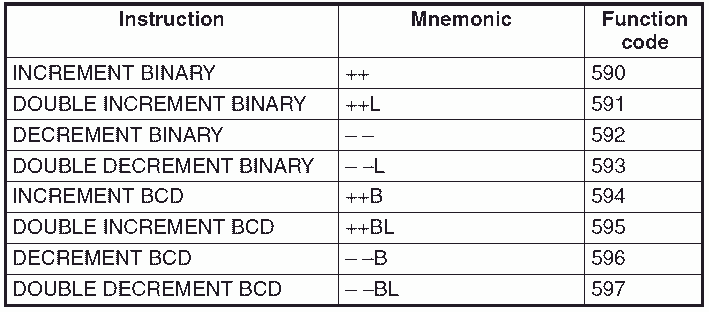These instructions are useful for indirect addressing.

INCREMENT BCD: ++B(594)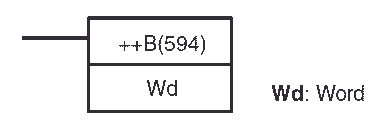This instruction will increment the selected word by 1. If the result is 0000 (9999 + 1) then the carry flag will be set.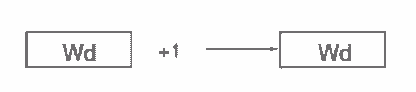In the following example you will see the use of the carry flag. We will use it to execute another BCD increment on the next register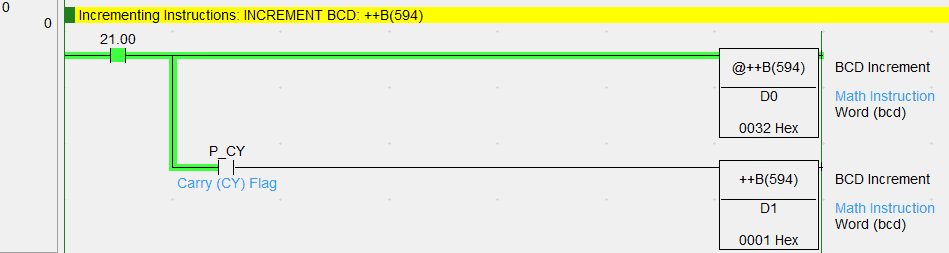DECREMENT BCD: – –B(596)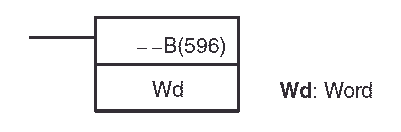This instruction will decrement the selected word by 1. If the result is 9999 (0000 – 1) then the carry flag will be set.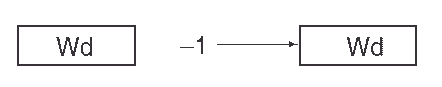The following example will demonstrate the use of the carry flag.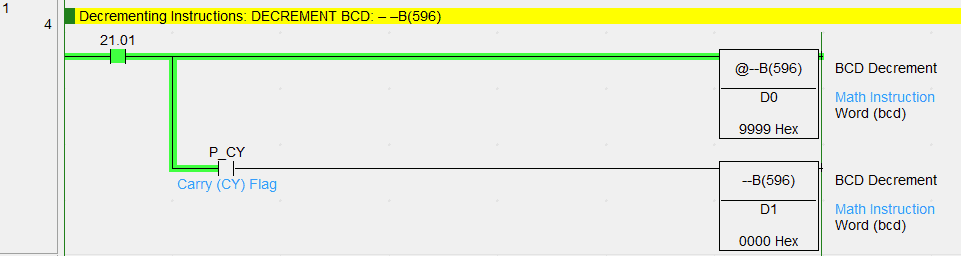Symbol Math Instructions
These instructions deal with binary or BCD addition, subtraction, multiplication and division. The word can be a signed (negative numbers) or unsigned value, single or double word.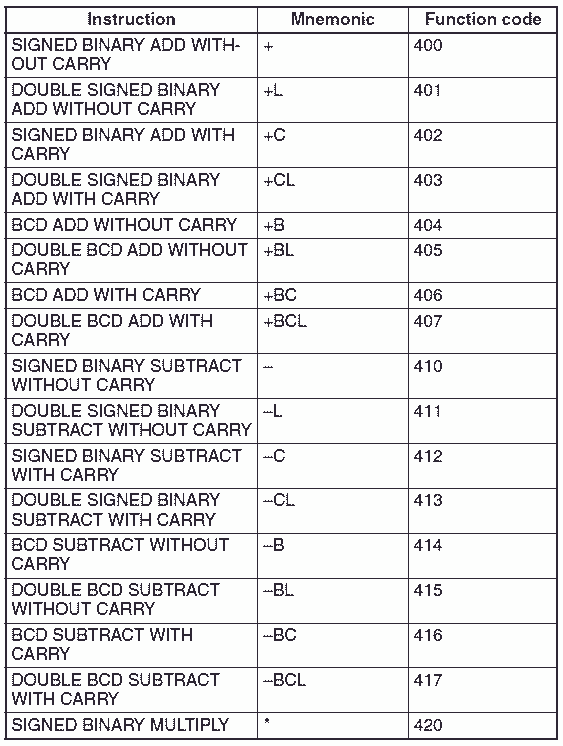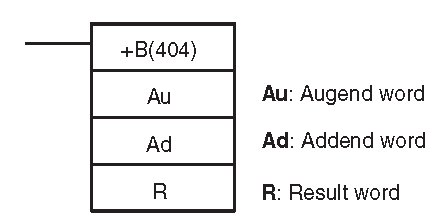This instruction will add two 16 bit words together and set the carry flag if the value is over 9999.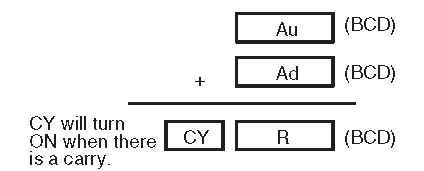In the following example we are using the BCD add without carry instruction. When the carry flag is set we use a BCD add with carry instruction. The BCD with carry instruction will add zero to the result word. So only the carry is added.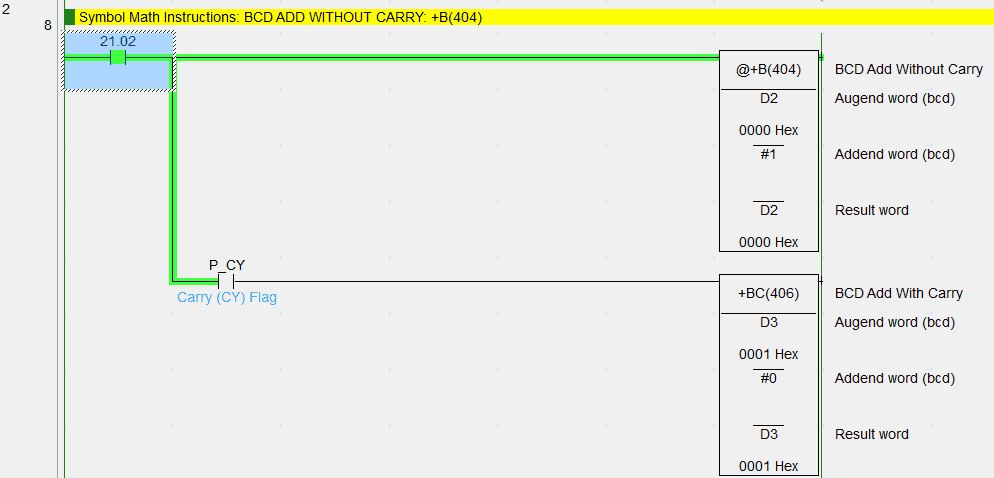BCD SUBTRACT WITHOUT CARRY: –B(414)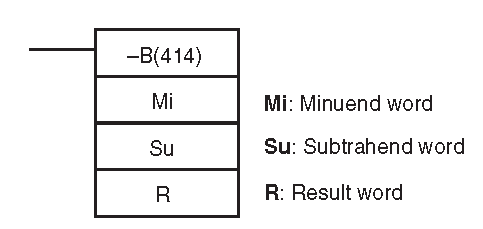This instruction will subtract two 16 bit words from each other and set the carry flag if the value is less than 0.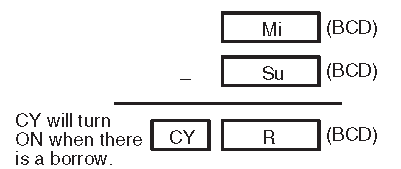This example will subtract the two words and set the carry flag. We then use the carry flag to do a BCD subtract with the carry flag to demonstrate the use of the instruction.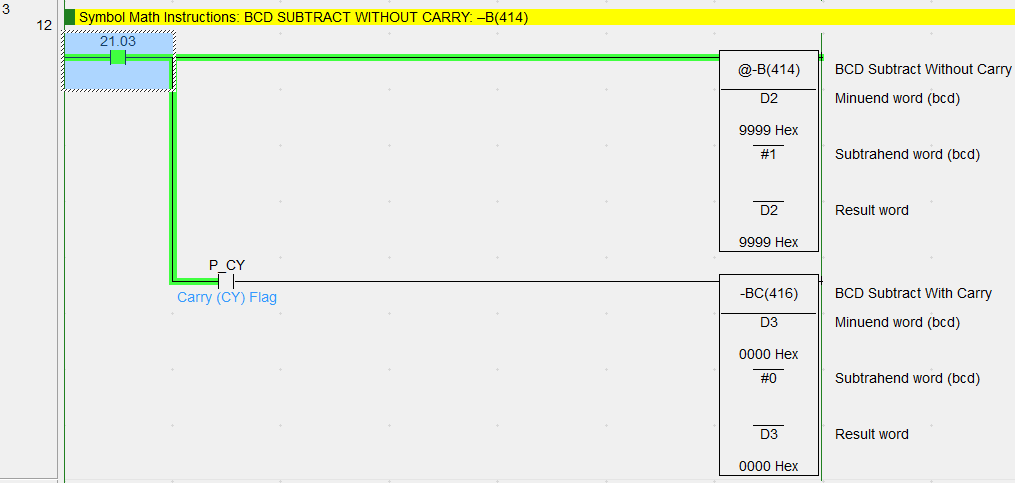BCD MULTIPLY: *B(424)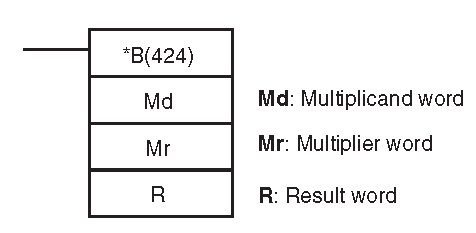This instruction will multiply two 16 bit words together and the result will be placed in two 16 bit words.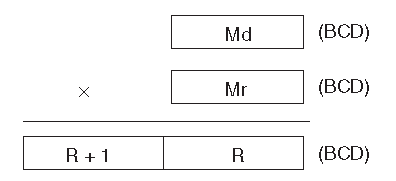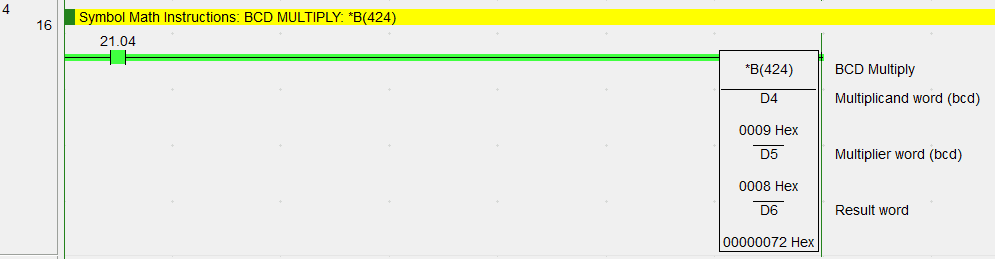BCD DIVIDE: /B(434)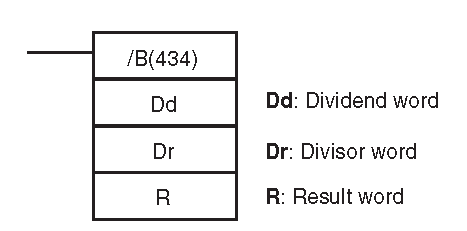This instruction will divide two 16 bit words and the result word will have the answer. The second result word will have the remainder.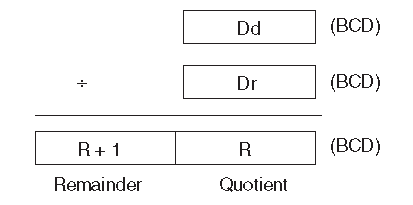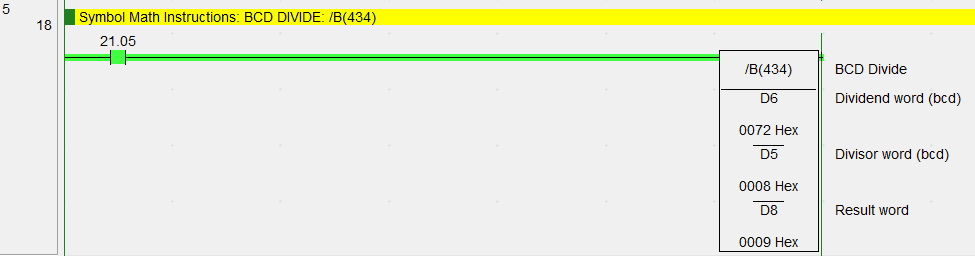Special Math Instructions
These instructions are used to find the square root of a BCD or binary number, floating point divide, arithmetic process or a bit counter.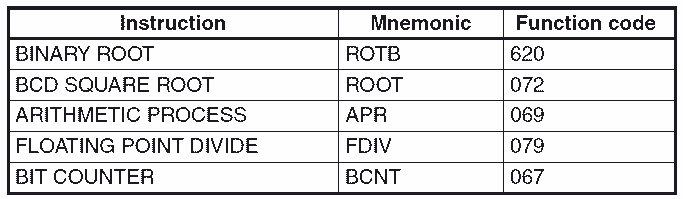BIT COUNTER: BCNT(067)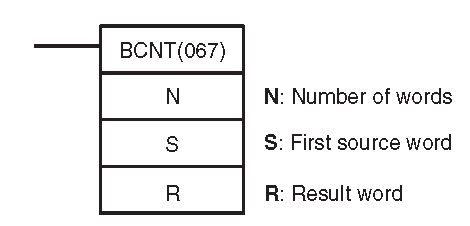The bit counter will count all of the bits in the words specified. The result word will contain the hexadecimal value of the number.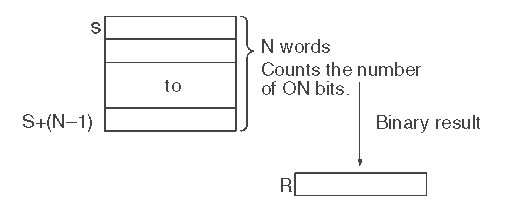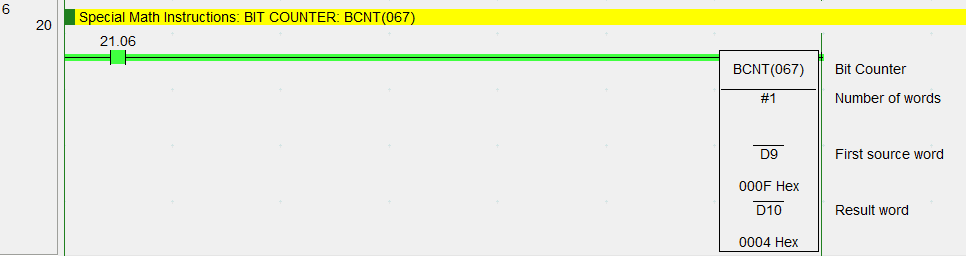Floating Point Math Instructions
The floating point instructions will convert data and perform floating point arithmetic operations. Floating point is used for precision calculations. The range of values that can be set for the 32 bit (2 words) single floating point is -3.4E+38 to +3.4E+38. The following table shows all of the calculations and conversions that can be done.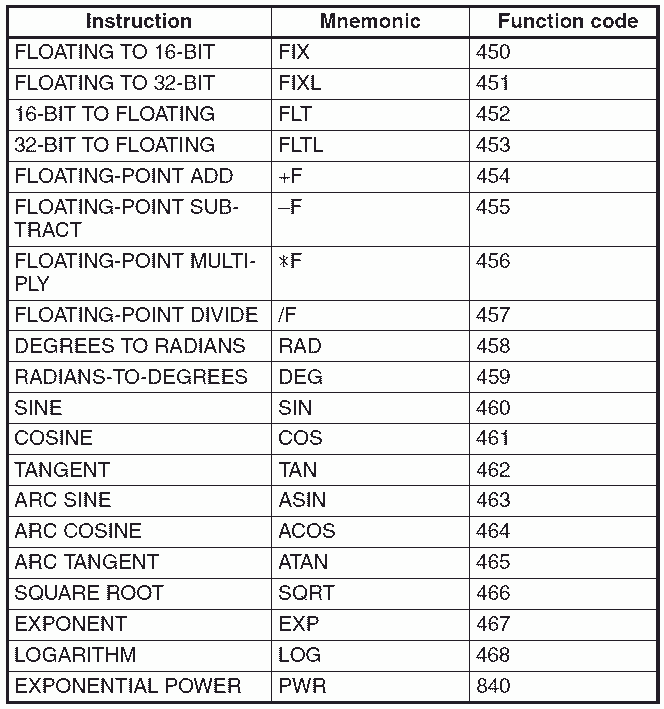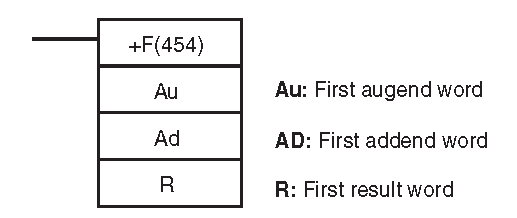This will add two 32 bit floating point data together and put the result in a 32 point floating point. Online floating point converts can help in determining your numbers. http://www.binaryconvert.com/convert_float.html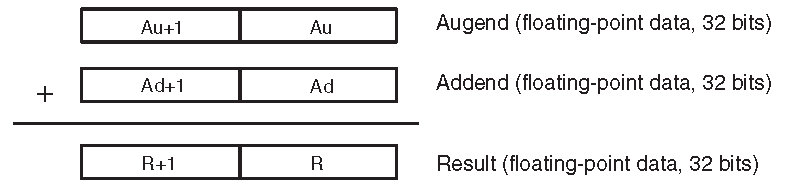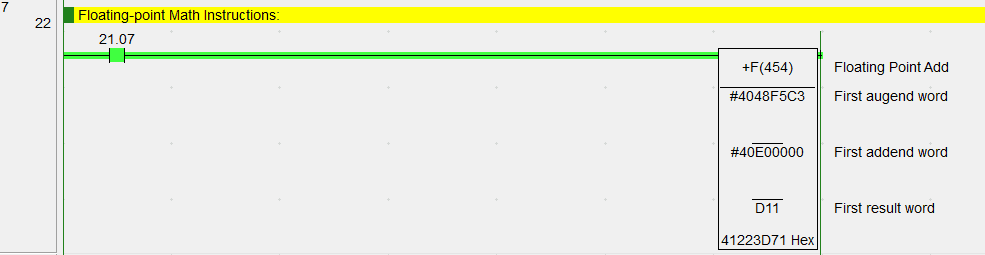Double Floating Point Math Instructions
The double floating point instructions will convert data and perform double floating point arithmetic operations. Double floating point is used for precision calculations. The range of values that can be set for the 64 bit (4 words) single floating point is -1.7E+308 to +1.7E+308. The following table shows all of the calculations and conversions that can be done.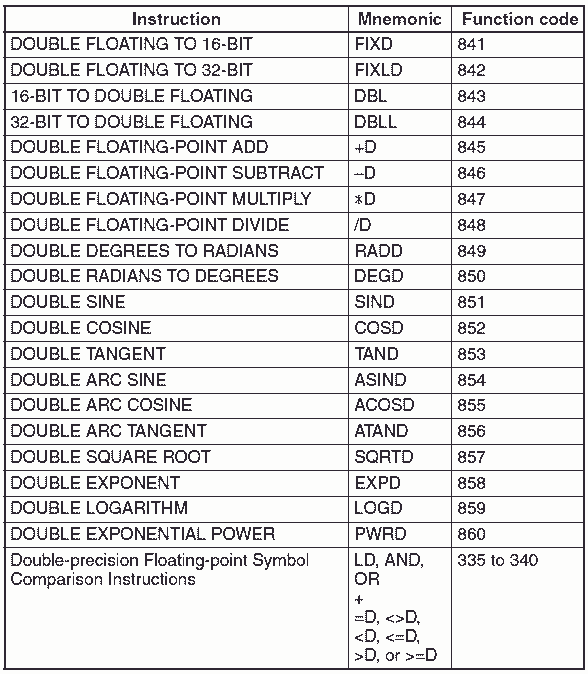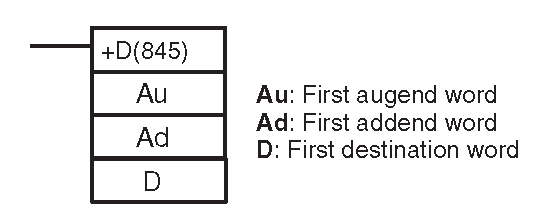This will add two 32 bit floating point data together and put the result in a 32 point floating point. Online floating point converts can help in determining your numbers.
http://www.binaryconvert.com/convert_double.html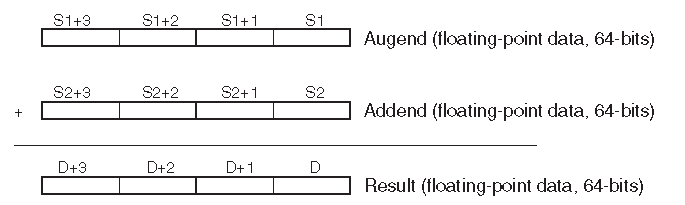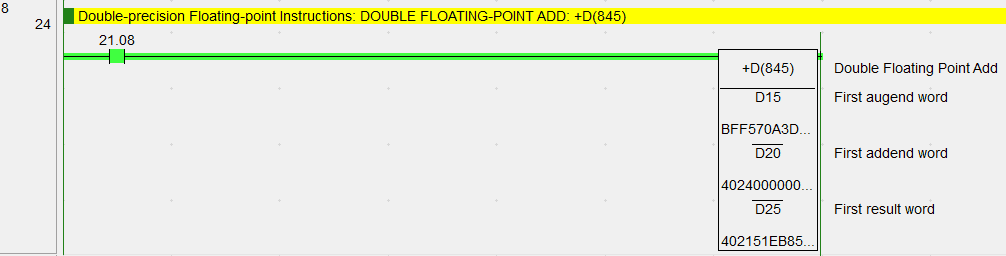You can see that we have many different math instructions in the Omron CP1H. This should give you a good idea of what is possible.

See the YouTube video below on math instructions in the Omron CP1H PLC.

The following is a list of manuals associated with the CP1H programmable logic controller. See the descriptions for each of these manuals in the first post: Omron CP1H System Hardware
W450 – SYSMAC CP Series CP1H CPU Unit Operation Manual
W451 – SYSMAC CP Series CP1H CPU Unit Programming Manual
W342 – SYSMAC CS/CJ series Communications Commands Reference Manual
W446 – SYSMAC CX-Programmer Ver. 6.1 Operation Manual
W447 – SYSMAC CX-Programmer Ver. 6.1 Operation Manual Function Blocks
W444 – CX-One FA Integrated Tool Package Setup Manual
W445 – CX-Integrator Operation Manual
W344 – CX-Protocol Operation Manual

Next time we will look at data conversion instructions in the Omron CP1H PLC.

Watch on YouTube : Omron CP1H Math Instructions
Thank you,
Garry

If you’re like most of my readers, you’re committed to learning about technology. Numbering systems used in PLC’s are not difficult to learn and understand. We will walk through the numbering systems used in PLCs. This includes Bits, Decimal, Hexadecimal, ASCII and Floating Point.

To get this free article, subscribe to my free email newsletter.

Use the information to inform other people how numbering systems work. Sign up now.